Resources Section

Web Application for Symmetrical Componets: http://personal.strath.ac.uk/steven.m.blair/seq/

Do you have a question? Click on the "Questions & Answers forum" and ask away!

This video was brought to you by GeneralPAC.com, making power systems Intuitive, Open and Free for Everyone, Everywhere. Consider subscribing and supporting through patreon.com/GeneralPAC. This is a mechanism for you to support us financially so we can continue making high quality power system video tutorials. Our corporate sponsor for this topic is AllumiaX.com from Seattle, Washington. Contact them for industrial and commercial power system studies.

Principals of Symmetrical Components Part 4B

In this part we're going to do a mathematical example of the breakdown of the system phaser to get the positive sequence component, negative sequence component and zero sequence component. Before we get into it we should clarify exactly what's going on here, so we have a very good picture in our heads. First of all the red phaser is IA, so think of this as phase A current. The yellow phaser is phase B current, and the blue phaser is phase C current. If they all rotated in the counterclockwise direction then they would make an A, B, C phase rotation. So our system phaser has an A, B, C phase rotation.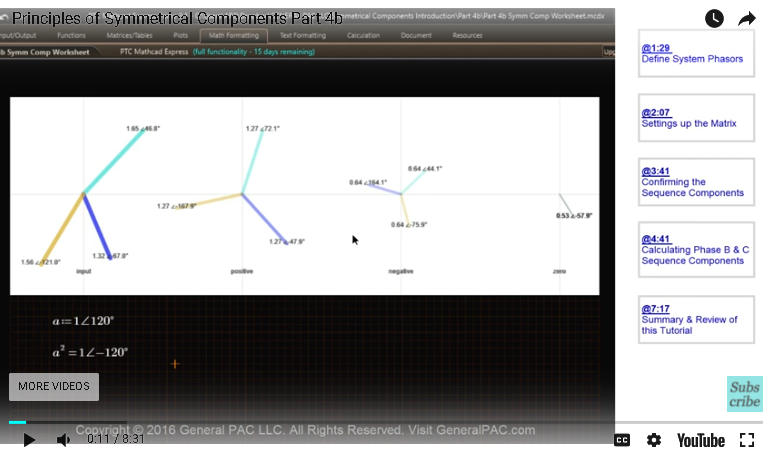The positive sequence component also has a phase rotation of the system phaser, so it also has an A, B, C phase rotation. The negative sequence component is always the opposite as the system phaser. So we can expect this to be an A, C, B phase rotation. The zero sequence component, although they all rotate in the counterclockwise direction, same as the negative and positive sequence component as well as the system phaser, the zero sequence component doesn't necessarily have a phase sequence. All three phasers have equal magnitude, and they all have the same phase angle, but they overlap each other. So keep that in mind.

So, first of all we're going to say that IA, which is the red phaser here, that is equal to 1.65 at the angle of 46.8 degrees. Then we're going to say that IB, which is the yellow phaser, that is equal to 1.32 at -67 degrees and IC, which is the blue phaser is 1.56 at -121 degrees. Okay, so there we have it. So I'm going rearrange this so we have more room to work with. So in part four we learned of the matrix to calculate the positive and negative and zero sequence components based off the system phasers. So we're going to input a 1 x 3 matrix first. This is going to be our zero sequence component, our positive sequence component and our negative sequence component for phase A. And that is equal to a 3 x 3 matrix, which we had defined as a inverse in the previous tutorial. So, it's going to look something like that.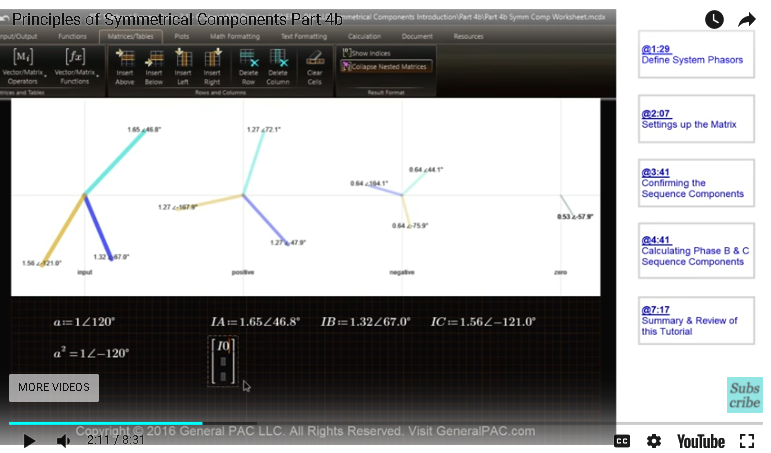And then we have to multiply this entire matrix by a constant of one-third and then lastly we have to multiply the A inverse by the original system phaser, which a 1 x 3 matrix, which equals A, B and C system phaser. So when we calculate this we get something like this. So our zero sequence component for phase A, which is right there, it's not correct. So let's figure out how we messed up. So our zero positive and negative sequence component for phase A does not match up with what the answer should be. So let's see. We probably defined this, one of these guys incorrectly. So IA was equal to 1.65 at 46.8 degrees, IB was equal to 1.32 at, looks like there's a negative sign right there. So we missed a negative sign here. So let's put in a negative sign and see what happens. And there you go. Okay, so now they are all matching up. I just missed the negative sign right there which messed up the entire problem.

But what we see here is that sequence component is equal to 0.53 at -57.9 degrees, which is very similar to what we got. The positive sequence component for phase A or for our reference phaser is equal to 1.27 at 72.13 degrees, 1.27 at 72.1 degrees and then our negative sequence component for our reference phaser is equal to 0.64 at 44.1 degrees, which makes sense. Now it's very, very important to note that when we do this, when we take the inverse of the A matrix and we multiply everything to get the positive, negative and zero sequence component what we get is our reference phasers, which we define as phase A. So we get zero sequence component for phase A, positive sequence component for phase A and negative sequence component for phase A. If we want to calculate the rest of the phases for the symmetrical components here's what we have to do.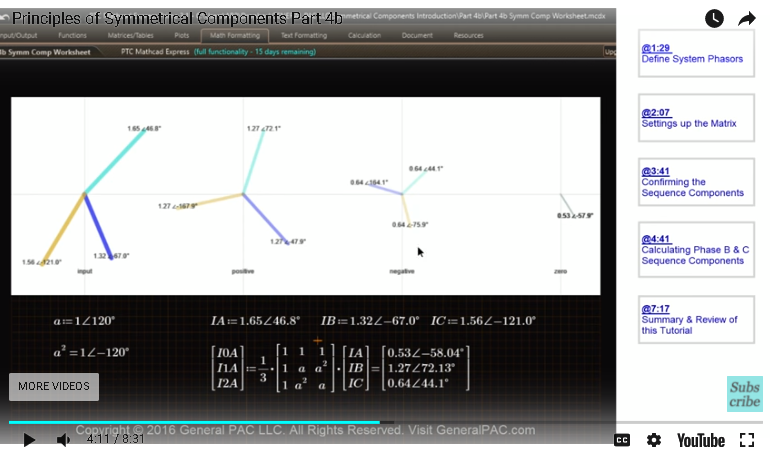Let's start with zero sequence component. We know that the zero sequence component is equal to that. And we know by principals of symmetrical component that the zero sequence component for phase B is going to equal the zero sequence component for phase A because they're all equal to each other. Similarly this is what we're going to get for phase C. So this is what we get for zero sequence component for phase A, B and C. They all have the same magnitude and they all have the same phase angle. Then for positive sequence component this is what we get, 1.27 at 72.13 degrees. This one matches this one, so positive sequence component for phase A. So this is phase A and then this yellow here is phase B. So if we wanted to calculate phase B while we could simply take phase A and then rotate it 240 degrees. So we can multiply it by the A superscript 2 operator and this is what we get, 1.27 at 47.9 degrees, which matches our positive sequence component for phase B.

Similarly for phase C we could take the positive sequence and rotate it by 120 degrees and we get 1.27 at -167.9 degrees. So for the negative sequence component this is what we calculated, 0.64 at 44.1 degrees, for the negative sequence component for phase A. Now if you wanted to calculate the negative sequence component for phase B we just take phase A right here. So here's phase A and here's phase B, so we just take phase A and then we multiply it by the A operator which would effectively rotate this by 120 degrees and here's what we get, 0.64 at 164.1 degrees, which matches up. Then similarly for the negative sequence component phase C, which is the blue, we had to take phase A and rotate it 240 degrees. We take phase A and then rotate it by 240 degrees and here's what we get, 0.64 at -75.9 degrees.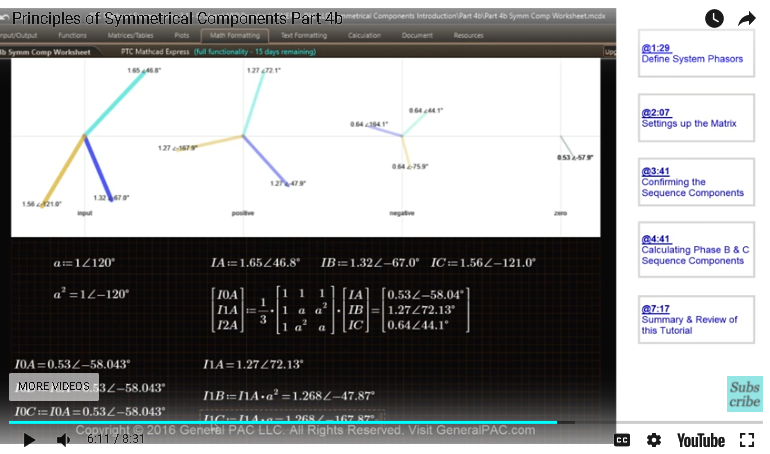There you have it. So, in this tutorial all we did was we defined our system phasers, IA, IB and IC, and we use our A inverse matrix, which is 1/3 x this matrix here and we multiply that by our system phasers, IA x IB x IC, and what we got was the zero sequence component for phase A, positive sequence component for phase A and negative sequence component for phase A. Now, it's important to remember that these here are symmetrical components for phase A. To get phase B and C we have to rotate phase A accordingly based off the principals of symmetrical components and get phase B and phase C symmetrical components that way.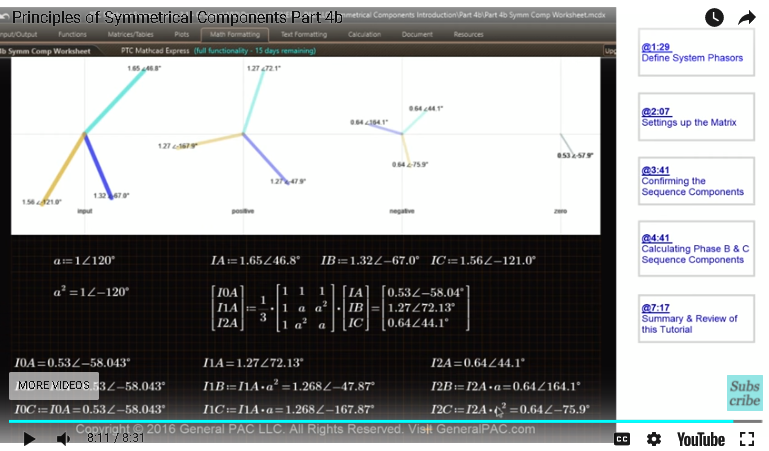This concludes our tutorial. Now if you have any questions there is a forum link on the bottom of this video. Please click on that and ask away on the forum. If you haven't subscribed already please click on the bottom right corner of the screen to subscribe to this channel. This video was brought to you by generalpac.com, Making Power Systems Intuitive.

Greetings from the GeneralPAC Team!

We make high-quality Power Systems Video Tutorials on complex topics that are free and open to everyone!  Thank you so much for supporting us through Patreon so can continue doing good and valuable work.

What is Patreon and why do we use it?

Patreon is a fantastic portal that allows our fans and community to make monthly contribution (like Netflix subscription) so we can continue creating high-quality power systems video tutorials. In return, you get access to incredible perks like voting on future topics, getting your questions answered, access to VIP Q/A webinars with the creators of GeneralPAC, and much more! We THANK YOU for supporting us

Why do we need your support?

An incredible amount of time and effort is needed to develop high-quality video tutorials. Each video (Part 1 for example) takes approximately 10 hours to complete which includes learning the concept ourselves, brainstorming creative ways to teach and explain the concepts, writing the script, audio recording, video recording, and editing. It's no wonder why Hundreds-of-Thousands of people have watched, liked, subscribed, and left positive comments on Youtube channel. Your support truly makes all the difference.